# 10.4 Polar coordinates: graphs  (Page 7/16)

 Page 7 / 16

Sketch the graph of $\text{\hspace{0.17em}}r=-\theta \text{\hspace{0.17em}}$ over the interval $\text{\hspace{0.17em}}\left[0,4\pi \right].$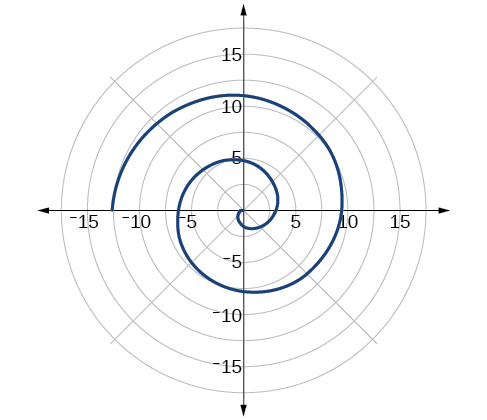## Summary of curves

We have explored a number of seemingly complex polar curves in this section. [link] and [link] summarize the graphs and equations for each of these curves.

Access these online resources for additional instruction and practice with graphs of polar coordinates.

## Key concepts

• It is easier to graph polar equations if we can test the equations for symmetry with respect to the line $\text{\hspace{0.17em}}\theta =\frac{\pi }{2},\text{\hspace{0.17em}}$ the polar axis, or the pole.
• There are three symmetry tests that indicate whether the graph of a polar equation will exhibit symmetry. If an equation fails a symmetry test, the graph may or may not exhibit symmetry. See [link] .
• Polar equations may be graphed by making a table of values for $\text{\hspace{0.17em}}\theta \text{\hspace{0.17em}}$ and $\text{\hspace{0.17em}}r.$
• The maximum value of a polar equation is found by substituting the value $\text{\hspace{0.17em}}\theta \text{\hspace{0.17em}}$ that leads to the maximum value of the trigonometric expression.
• The zeros of a polar equation are found by setting $\text{\hspace{0.17em}}r=0\text{\hspace{0.17em}}$ and solving for $\text{\hspace{0.17em}}\theta .\text{\hspace{0.17em}}$ See [link] .
• Some formulas that produce the graph of a circle in polar coordinates are given by $\text{\hspace{0.17em}}r=a\mathrm{cos}\text{\hspace{0.17em}}\theta \text{\hspace{0.17em}}$ and $\text{\hspace{0.17em}}r=a\mathrm{sin}\text{\hspace{0.17em}}\theta .\text{\hspace{0.17em}}$ See [link] .
• The formulas that produce the graphs of a cardioid are given by $\text{\hspace{0.17em}}r=a±b\mathrm{cos}\text{\hspace{0.17em}}\theta \text{\hspace{0.17em}}$ and $\text{\hspace{0.17em}}r=a±b\mathrm{sin}\text{\hspace{0.17em}}\theta ,\text{\hspace{0.17em}}$ for $\text{\hspace{0.17em}}a>0,\text{\hspace{0.17em}}\text{\hspace{0.17em}}b>0,\text{\hspace{0.17em}}$ and $\text{\hspace{0.17em}}\frac{a}{b}=1.\text{\hspace{0.17em}}$ See [link] .
• The formulas that produce the graphs of a one-loop limaçon are given by $\text{\hspace{0.17em}}r=a±b\mathrm{cos}\text{\hspace{0.17em}}\theta \text{\hspace{0.17em}}$ and $\text{\hspace{0.17em}}r=a±b\mathrm{sin}\text{\hspace{0.17em}}\theta \text{\hspace{0.17em}}$ for $\text{\hspace{0.17em}}1<\frac{a}{b}<2.\text{\hspace{0.17em}}$ See [link] .
• The formulas that produce the graphs of an inner-loop limaçon are given by $\text{\hspace{0.17em}}r=a±b\mathrm{cos}\text{\hspace{0.17em}}\theta \text{\hspace{0.17em}}$ and $\text{\hspace{0.17em}}r=a±b\mathrm{sin}\text{\hspace{0.17em}}\theta \text{\hspace{0.17em}}$ for $\text{\hspace{0.17em}}a>0,\text{\hspace{0.17em}}\text{\hspace{0.17em}}b>0,\text{\hspace{0.17em}}$ and $\text{\hspace{0.17em}}a See [link] .
• The formulas that produce the graphs of a lemniscates are given by $\text{\hspace{0.17em}}{r}^{2}={a}^{2}\mathrm{cos}\text{\hspace{0.17em}}2\theta \text{\hspace{0.17em}}$ and $\text{\hspace{0.17em}}{r}^{2}={a}^{2}\mathrm{sin}\text{\hspace{0.17em}}2\theta ,\text{\hspace{0.17em}}$ where $\text{\hspace{0.17em}}a\ne 0.$ See [link] .
• The formulas that produce the graphs of rose curves are given by $\text{\hspace{0.17em}}r=a\mathrm{cos}\text{\hspace{0.17em}}n\theta \text{\hspace{0.17em}}$ and $\text{\hspace{0.17em}}r=a\mathrm{sin}\text{\hspace{0.17em}}n\theta ,\text{\hspace{0.17em}}$ where $\text{\hspace{0.17em}}a\ne 0;\text{\hspace{0.17em}}$ if $\text{\hspace{0.17em}}n\text{\hspace{0.17em}}$ is even, there are $\text{\hspace{0.17em}}2n\text{\hspace{0.17em}}$ petals, and if $\text{\hspace{0.17em}}n\text{\hspace{0.17em}}$ is odd, there are $\text{\hspace{0.17em}}n\text{\hspace{0.17em}}$ petals. See [link] and [link] .
• The formula that produces the graph of an Archimedes’ spiral is given by $\text{\hspace{0.17em}}r=\theta ,\text{\hspace{0.17em}}\text{\hspace{0.17em}}\theta \ge 0.\text{\hspace{0.17em}}$ See [link] .

## Verbal

Describe the three types of symmetry in polar graphs, and compare them to the symmetry of the Cartesian plane.

Symmetry with respect to the polar axis is similar to symmetry about the $\text{\hspace{0.17em}}x$ -axis, symmetry with respect to the pole is similar to symmetry about the origin, and symmetric with respect to the line $\text{\hspace{0.17em}}\theta =\frac{\pi }{2}\text{\hspace{0.17em}}$ is similar to symmetry about the $\text{\hspace{0.17em}}y$ -axis.

Which of the three types of symmetries for polar graphs correspond to the symmetries with respect to the x -axis, y -axis, and origin?

What are the steps to follow when graphing polar equations?

Test for symmetry; find zeros, intercepts, and maxima; make a table of values. Decide the general type of graph, cardioid, limaçon, lemniscate, etc., then plot points at and sketch the graph.

Describe the shapes of the graphs of cardioids, limaçons, and lemniscates.

What part of the equation determines the shape of the graph of a polar equation?

The shape of the polar graph is determined by whether or not it includes a sine, a cosine, and constants in the equation.

## Graphical

For the following exercises, test the equation for symmetry.

$r=5\mathrm{cos}\text{\hspace{0.17em}}3\theta$

$r=3-3\mathrm{cos}\text{\hspace{0.17em}}\theta$

symmetric with respect to the polar axis

$r=3+2\mathrm{sin}\text{\hspace{0.17em}}\theta$

$r=3\mathrm{sin}\text{\hspace{0.17em}}2\theta$

symmetric with respect to the polar axis, symmetric with respect to the line $\theta =\frac{\pi }{2},$ symmetric with respect to the pole

$r=4$

$r=2\theta$

no symmetry

$r=4\mathrm{cos}\text{\hspace{0.17em}}\frac{\theta }{2}$

$r=\frac{2}{\theta }$

no symmetry

$r=3\sqrt{1-{\mathrm{cos}}^{2}\theta }$

$r=\sqrt{5\mathrm{sin}\text{\hspace{0.17em}}2\theta }$

symmetric with respect to the pole

For the following exercises, graph the polar equation. Identify the name of the shape.

$r=3\mathrm{cos}\text{\hspace{0.17em}}\theta$

$r=4\mathrm{sin}\text{\hspace{0.17em}}\theta$

circle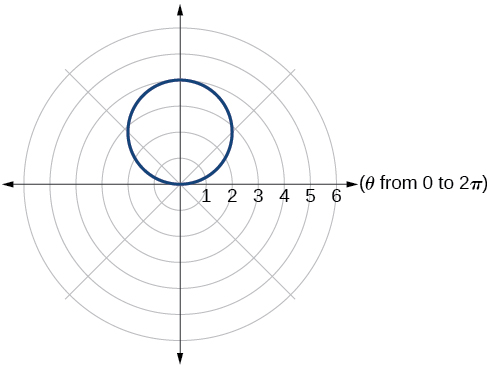$r=2+2\mathrm{cos}\text{\hspace{0.17em}}\theta$

$r=2-2\mathrm{cos}\text{\hspace{0.17em}}\theta$

cardioid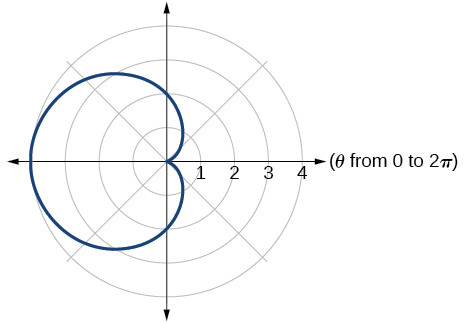$r=5-5\mathrm{sin}\text{\hspace{0.17em}}\theta$

$r=3+3\mathrm{sin}\text{\hspace{0.17em}}\theta$

cardioid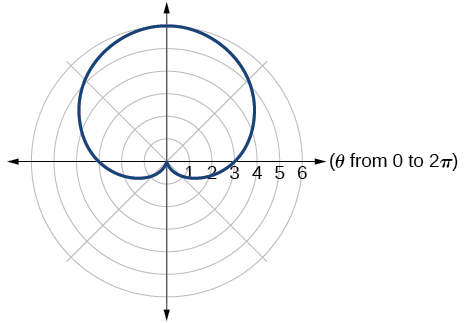$r=3+2\mathrm{sin}\text{\hspace{0.17em}}\theta$

$r=7+4\mathrm{sin}\text{\hspace{0.17em}}\theta$

one-loop/dimpled limaçon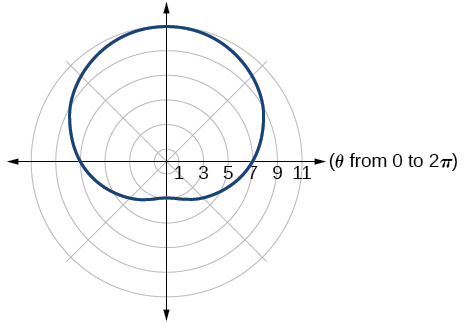$r=4+3\mathrm{cos}\text{\hspace{0.17em}}\theta$

$r=5+4\mathrm{cos}\text{\hspace{0.17em}}\theta$

one-loop/dimpled limaçon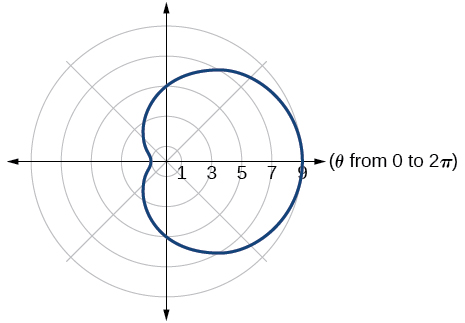$r=10+9\mathrm{cos}\text{\hspace{0.17em}}\theta$

$r=1+3\mathrm{sin}\text{\hspace{0.17em}}\theta$

inner loop/two-loop limaçon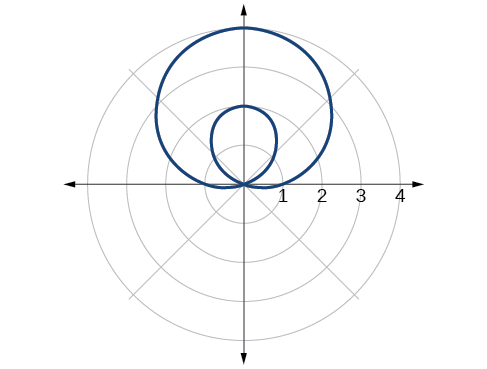$r=2+5\mathrm{sin}\text{\hspace{0.17em}}\theta$

$r=5+7\mathrm{sin}\text{\hspace{0.17em}}\theta$

inner loop/two-loop limaçon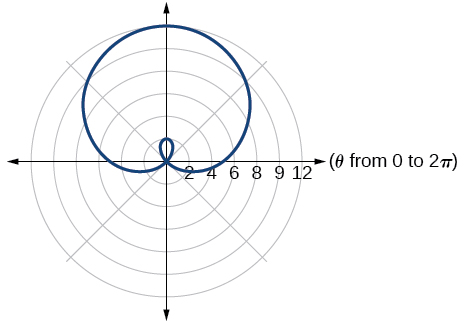$r=2+4\mathrm{cos}\text{\hspace{0.17em}}\theta$

$r=5+6\mathrm{cos}\text{\hspace{0.17em}}\theta$

inner loop/two-loop limaçon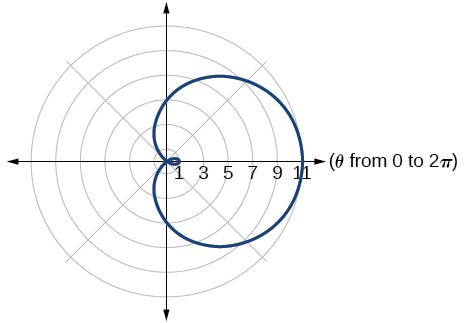${r}^{2}=36\mathrm{cos}\left(2\theta \right)$

${r}^{2}=10\mathrm{cos}\left(2\theta \right)$

lemniscate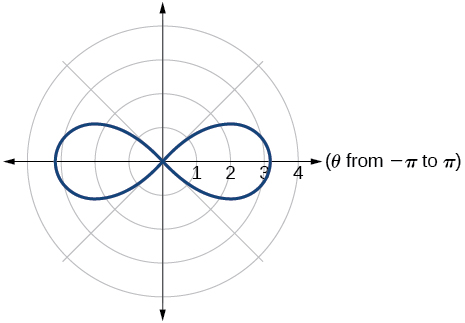${r}^{2}=4\mathrm{sin}\left(2\theta \right)$

${r}^{2}=10\mathrm{sin}\left(2\theta \right)$

lemniscate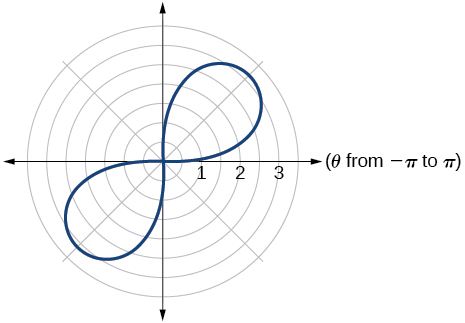$r=3\text{sin}\left(2\theta \right)$

$r=3\text{cos}\left(2\theta \right)$

rose curve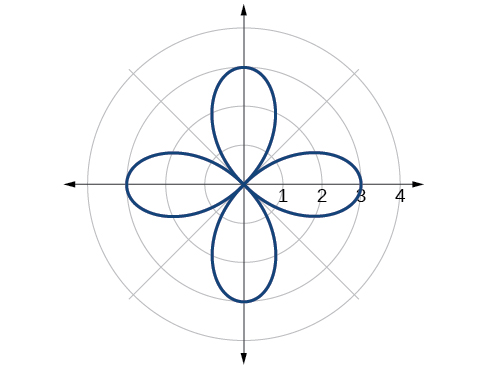$r=5\text{sin}\left(3\theta \right)$

$r=4\text{sin}\left(4\theta \right)$

rose curve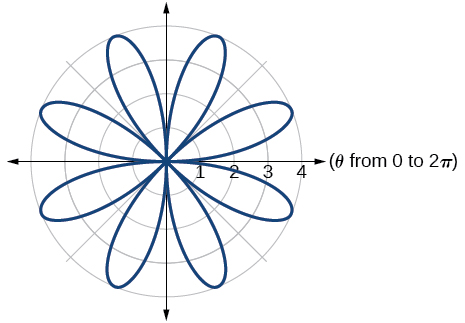$r=4\text{sin}\left(5\theta \right)$

$r=-\theta$

Archimedes’ spiral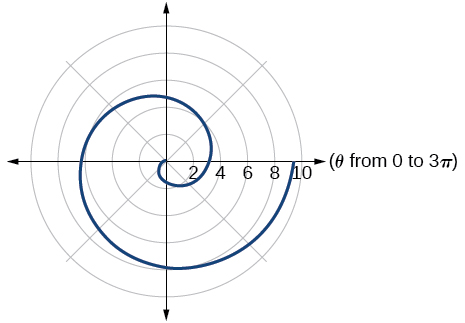$r=2\theta$

$r=-3\theta$

Archimedes’ spiral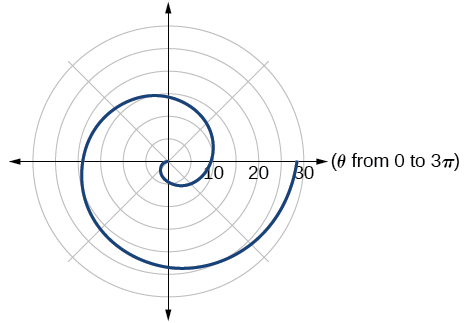## Technology

For the following exercises, use a graphing calculator to sketch the graph of the polar equation.

$r=\frac{1}{\theta }$

$r=\frac{1}{\sqrt{\theta }}$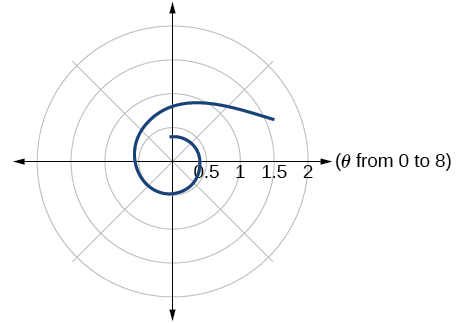$r=2\mathrm{sin}\text{\hspace{0.17em}}\theta \mathrm{tan}\text{\hspace{0.17em}}\theta ,$ a cissoid

$r=2\sqrt{1-{\mathrm{sin}}^{2}\theta }$ , a hippopede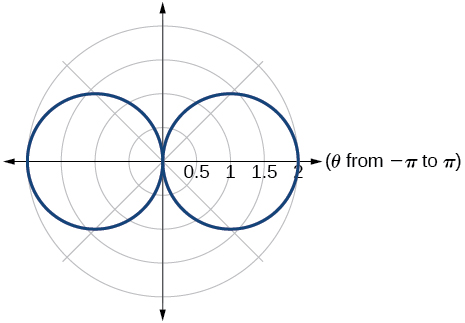$r=5+\mathrm{cos}\left(4\theta \right)$

$r=2-\mathrm{sin}\left(2\theta \right)$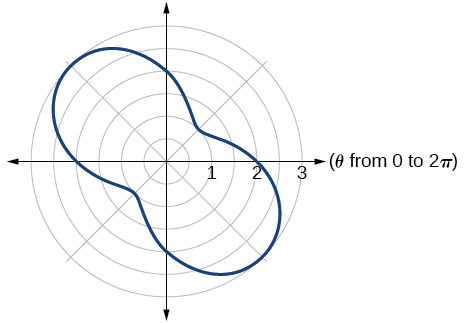$r={\theta }^{2}$

$r=\theta +1$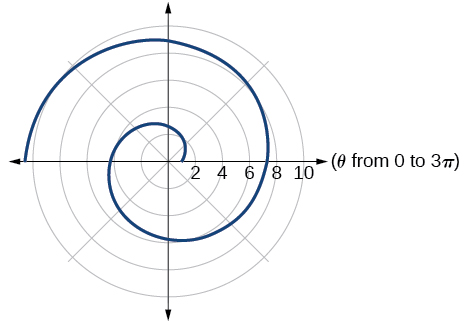$r=\theta \mathrm{sin}\text{\hspace{0.17em}}\theta$

$r=\theta \mathrm{cos}\text{\hspace{0.17em}}\theta$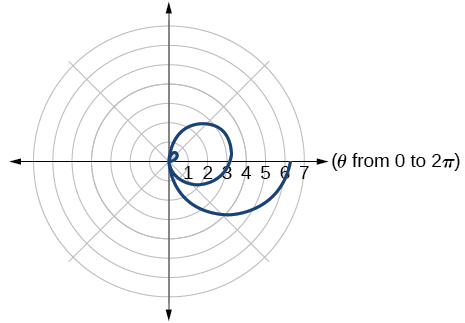For the following exercises, use a graphing utility to graph each pair of polar equations on a domain of $\text{\hspace{0.17em}}\left[0,4\pi \right]\text{\hspace{0.17em}}$ and then explain the differences shown in the graphs.

$r=\theta ,r=-\theta$

$r=\theta ,r=\theta +\mathrm{sin}\text{\hspace{0.17em}}\theta$

They are both spirals, but not quite the same.

$r=\mathrm{sin}\text{\hspace{0.17em}}\theta +\theta ,r=\mathrm{sin}\text{\hspace{0.17em}}\theta -\theta$

$r=2\mathrm{sin}\left(\frac{\theta }{2}\right),r=\theta \mathrm{sin}\left(\frac{\theta }{2}\right)$

Both graphs are curves with 2 loops. The equation with a coefficient of $\text{\hspace{0.17em}}\theta \text{\hspace{0.17em}}$ has two loops on the left, the equation with a coefficient of 2 has two loops side by side. Graph these from 0 to $\text{\hspace{0.17em}}4\pi \text{\hspace{0.17em}}$ to get a better picture.

$r=\mathrm{sin}\left(\mathrm{cos}\left(3\theta \right)\right)\text{\hspace{0.17em}}\text{\hspace{0.17em}}r=\mathrm{sin}\left(3\theta \right)$

On a graphing utility, graph $\text{\hspace{0.17em}}r=\mathrm{sin}\left(\frac{16}{5}\theta \right)\text{\hspace{0.17em}}$ on $\text{\hspace{0.17em}}\left[0,4\pi \right],\left[0,8\pi \right],\left[0,12\pi \right],\text{\hspace{0.17em}}$ and $\text{\hspace{0.17em}}\left[0,16\pi \right].\text{\hspace{0.17em}}$ Describe the effect of increasing the width of the domain.

When the width of the domain is increased, more petals of the flower are visible.

On a graphing utility, graph and sketch $\text{\hspace{0.17em}}r=\mathrm{sin}\text{\hspace{0.17em}}\theta +{\left(\mathrm{sin}\left(\frac{5}{2}\theta \right)\right)}^{3}\text{\hspace{0.17em}}$ on $\text{\hspace{0.17em}}\left[0,4\pi \right].$

On a graphing utility, graph each polar equation. Explain the similarities and differences you observe in the graphs.

$\begin{array}{l}\begin{array}{l}\\ {r}_{1}=3\mathrm{sin}\left(3\theta \right)\end{array}\hfill \\ {r}_{2}=2\mathrm{sin}\left(3\theta \right)\hfill \\ {r}_{3}=\mathrm{sin}\left(3\theta \right)\hfill \end{array}$

The graphs are three-petal, rose curves. The larger the coefficient, the greater the curve’s distance from the pole.

On a graphing utility, graph each polar equation. Explain the similarities and differences you observe in the graphs.

$\begin{array}{l}\begin{array}{l}\\ {r}_{1}=3+3\mathrm{cos}\text{\hspace{0.17em}}\theta \end{array}\hfill \\ {r}_{2}=2+2\mathrm{cos}\text{\hspace{0.17em}}\theta \hfill \\ {r}_{3}=1+\mathrm{cos}\text{\hspace{0.17em}}\theta \hfill \end{array}$

On a graphing utility, graph each polar equation. Explain the similarities and differences you observe in the graphs.

$\begin{array}{l}\begin{array}{l}\\ {r}_{1}=3\theta \end{array}\hfill \\ {r}_{2}=2\theta \hfill \\ {r}_{3}=\theta \hfill \end{array}$

The graphs are spirals. The smaller the coefficient, the tighter the spiral.

## Extensions

For the following exercises, draw each polar equation on the same set of polar axes, and find the points of intersection.

${r}_{1}=3+2\mathrm{sin}\text{\hspace{0.17em}}\theta ,\text{\hspace{0.17em}}{r}_{2}=2$

${r}_{1}=6-4\mathrm{cos}\text{\hspace{0.17em}}\theta ,\text{\hspace{0.17em}}{r}_{2}=4$

$\left(4,\frac{\pi }{3}\right),\left(4,\frac{5\pi }{3}\right)$

${r}_{1}=1+\mathrm{sin}\text{\hspace{0.17em}}\theta ,\text{\hspace{0.17em}}{r}_{2}=3\mathrm{sin}\text{\hspace{0.17em}}\theta$

${r}_{1}=1+\mathrm{cos}\text{\hspace{0.17em}}\theta ,\text{\hspace{0.17em}}{r}_{2}=3\mathrm{cos}\text{\hspace{0.17em}}\theta$

$\left(\frac{3}{2},\frac{\pi }{3}\right),\left(\frac{3}{2},\frac{5\pi }{3}\right)$

${r}_{1}=\mathrm{cos}\left(2\theta \right),\text{\hspace{0.17em}}{r}_{2}=\mathrm{sin}\left(2\theta \right)$

${r}_{1}={\mathrm{sin}}^{2}\left(2\theta \right),\text{\hspace{0.17em}}{r}_{2}=1-\mathrm{cos}\left(4\theta \right)$

$\left(0,\frac{\pi }{2}\right),\text{\hspace{0.17em}}\left(0,\pi \right),\text{\hspace{0.17em}}\left(0,\frac{3\pi }{2}\right),\text{\hspace{0.17em}}\left(0,2\pi \right)$

${r}_{1}=\sqrt{3},\text{\hspace{0.17em}}{r}_{2}=2\mathrm{sin}\left(\theta \right)$

${r}_{1}{}^{2}=\mathrm{sin}\text{\hspace{0.17em}}\theta ,{r}_{2}{}^{2}=\mathrm{cos}\text{\hspace{0.17em}}\theta$

$\left(\frac{\sqrt{8}}{2},\frac{\pi }{4}\right),\text{\hspace{0.17em}}\left(\frac{\sqrt{8}}{2},\frac{5\pi }{4}\right)\text{\hspace{0.17em}}$ and at $\text{\hspace{0.17em}}\theta =\frac{3\pi }{4},\text{\hspace{0.17em}}\text{\hspace{0.17em}}\frac{7\pi }{4}\text{\hspace{0.17em}}\text{\hspace{0.17em}}$ since $\text{\hspace{0.17em}}r\text{\hspace{0.17em}}$ is squared

${r}_{1}=1+\mathrm{cos}\text{\hspace{0.17em}}\theta ,\text{\hspace{0.17em}}{r}_{2}=1-\mathrm{sin}\text{\hspace{0.17em}}\theta$

f(x)=x/x+2 given g(x)=1+2x/1-x show that gf(x)=1+2x/3
proof
AUSTINE
sebd me some questions about anything ill solve for yall
how to solve x²=2x+8 factorization?
x=2x+8 x-2x=2x+8-2x x-2x=8 -x=8 -x/-1=8/-1 x=-8 prove: if x=-8 -8=2(-8)+8 -8=-16+8 -8=-8 (PROVEN)
Manifoldee
x=2x+8
Manifoldee
×=2x-8 minus both sides by 2x
Manifoldee
so, x-2x=2x+8-2x
Manifoldee
then cancel out 2x and -2x, cuz 2x-2x is obviously zero
Manifoldee
so it would be like this: x-2x=8
Manifoldee
then we all know that beside the variable is a number (1): (1)x-2x=8
Manifoldee
so we will going to minus that 1-2=-1
Manifoldee
so it would be -x=8
Manifoldee
so next step is to cancel out negative number beside x so we get positive x
Manifoldee
so by doing it you need to divide both side by -1 so it would be like this: (-1x/-1)=(8/-1)
Manifoldee
so -1/-1=1
Manifoldee
so x=-8
Manifoldee
Manifoldee
so we should prove it
Manifoldee
x=2x+8 x-2x=8 -x=8 x=-8 by mantu from India
mantu
lol i just saw its x²
Manifoldee
x²=2x-8 x²-2x=8 -x²=8 x²=-8 square root(x²)=square root(-8) x=sq. root(-8)
Manifoldee
I mean x²=2x+8 by factorization method
Kristof
I think x=-2 or x=4
Kristof
x= 2x+8 ×=8-2x - 2x + x = 8 - x = 8 both sides divided - 1 -×/-1 = 8/-1 × = - 8 //// from somalia
Mohamed
hii
Amit
how are you
Dorbor
well
Biswajit
can u tell me concepts
Gaurav
Find the possible value of 8.5 using moivre's theorem
which of these functions is not uniformly cintinuous on (0, 1)? sinx
which of these functions is not uniformly continuous on 0,1
solve this equation by completing the square 3x-4x-7=0
X=7
Muustapha
=7
mantu
x=7
mantu
3x-4x-7=0 -x=7 x=-7
Kr
x=-7
mantu
9x-16x-49=0 -7x=49 -x=7 x=7
mantu
what's the formula
Modress
-x=7
Modress
new member
siame
what is trigonometry
deals with circles, angles, and triangles. Usually in the form of Soh cah toa or sine, cosine, and tangent
Thomas
solve for me this equational y=2-x
what are you solving for
Alex
solve x
Rubben
you would move everything to the other side leaving x by itself. subtract 2 and divide -1.
Nikki
then I got x=-2
Rubben
it will b -y+2=x
Alex
goodness. I'm sorry. I will let Alex take the wheel.
Nikki
ouky thanks braa
Rubben
I think he drive me safe
Rubben
how to get 8 trigonometric function of tanA=0.5, given SinA=5/13? Can you help me?m
More example of algebra and trigo
What is Indices
If one side only of a triangle is given is it possible to solve for the unkown two sides?
cool
Rubben
kya
Khushnama
please I need help in maths
Okey tell me, what's your problem is?
NavinByByByByByByByByByBy